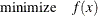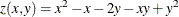An Unconstrained Optimization Example

An unconstrained optimization problem formulation is simplyFor example, suppose you wanted to find the minimum value of this polynomial:You can compactly specify and solve the optimization problem by using the OPTMODEL modeling language. Here is the program:

/* invoke procedure */
proc optmodel;
var x, y;  /* declare variables */

/* objective function */
min z=x**2 - x - 2*y - x*y + y**2;

/* now run the solver */
solve;

print x y;
quit;


This program produces the output in Figure 5.2.

Figure 5.2: Optimizing a Simple Polynomial

The OPTMODEL Procedure

Problem Summary
Objective Sense Minimization
Objective Function z

Number of Variables 2
Bounded Above 0
Bounded Below 0
Bounded Below and Above 0
Free 2
Fixed 0

Number of Constraints 0

Constraint Coefficients 0

Performance Information
Execution Mode Single-Machine

Solution Summary
Solver QP
Algorithm Interior Point
Objective Function z
Solution Status Optimal
Objective Value -2.333333333

Primal Infeasibility 0
Dual Infeasibility 6.861556E-17
Bound Infeasibility 0
Duality Gap 0
Complementarity 0

Iterations 0
Presolve Time 0.00
Solution Time 0.01

x y
1.3333 1.6667

In PROC OPTMODEL you specify the mathematical formulas that describe the behavior of the optimization problem that you want to solve. In the preceding example there were two independent variables in the polynomial,and. These are the optimization variables of the problem. In PROC OPTMODEL you declare optimization variables with the VAR statement. The formula that defines the quantity that you are seeking to optimize is called the objective function, or objective. The solver varies the values of the optimization variables when searching for an optimal value for the objective.

In the preceding example the objective function is named, declared with the MIN statement. The keyword MIN is an abbreviation for MINIMIZE. The expression that follows the equal sign (=) in the MIN statement defines the function to be minimized in terms of the optimization variables.

The VAR and MIN statements are just two of the many available PROC OPTMODEL declaration and programming statements. PROC OPTMODEL processes all such statements interactively, meaning that each statement is processed as soon as it is complete.

After PROC OPTMODEL has completed processing of declaration and programming statements, it processes the SOLVE statement, which submits the problem to a solver and prints a summary of the results. The PRINT statement displays the optimal values of the optimization variables x and y found by the solver.

It is worth noting that PROC OPTMODEL does not use a RUN statement but instead operates on an interactive basis throughout. You can continue to interact with PROC OPTMODEL even after invoking a solver. For example, you could modify the problem and issue another SOLVE statement (see the section Model Update).